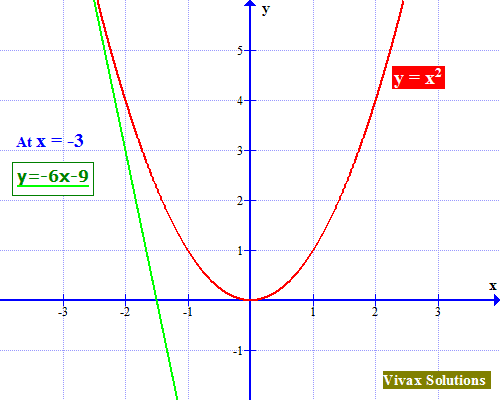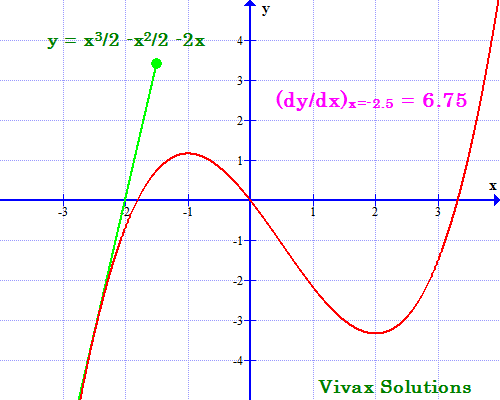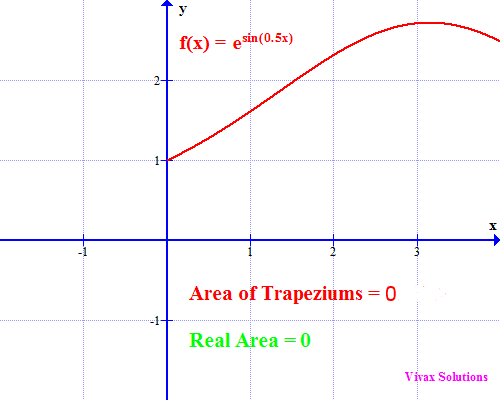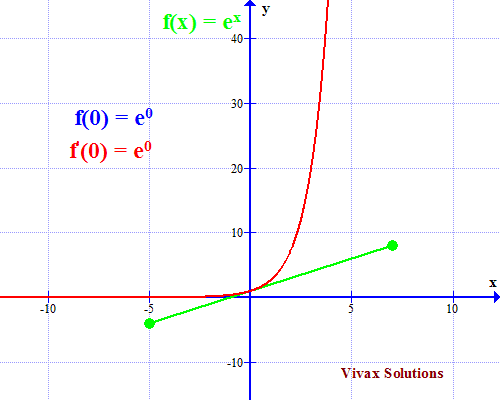### Maths LabMaths Lab consists of a few widely-used virtual mathematical tools. The quadratic equation generator, for instance, can produce thousands of questions - and at random - for you to practise - and all at a click of a button. Most of them, are easily factorable, which makes the experience in solving them a bit more pleasant.

Then, you have a programme to check whether the answers are correct. You can use the Quadratic Solver for that. Just type in the coefficient of x2, x and the constant to find the solutions.

There is a programme to generate simultaneous equations, number sequences and even the coordinates of straight lines. If you want to practise compound interest based calculations, there is a programme for that too.

The site is developed with Microsoft's .Net technology, ASP.Net, JavaScript, CSS and AJAX, in compliance with the guidelines of the W3C, the internet governing body.

A random equation for each click will appear here.

a =       b =       c =

#### Simultaneous Equation Generator

Two random equations will appear below, when clicked

#### Coordinates for a Straight Line Graph

Use the coordinates to find the equation of the line.

#### Compound Interest Calculator

P =    N =    R  =

#### Equations of tangents to a curve#### Exponential Functions### First Derivative - differential coefficient (dy/dx)#### The Trapezium Rule#### The Exponential FunctionMaths is challenging; so is finding the right book. K A Stroud, in this book, cleverly managed to make all the major topics crystal clear with plenty of examples; popularity of the book speak for itself - 7th edition in print.

### Recommended - GCSE & iGCSEThis is the best book available for the new GCSE(9-1) specification and iGCSE: there are plenty of worked examples; a really good collection of problems for practising; every single topic is adequately covered; the topics are organized in a logical order.

### Recommended for A LevelThis is the best book that can be recommended for the new A Level - Edexcel board: it covers every single topic in detail;lots of worked examples; ample problems for practising; beautifully and clearly presented.